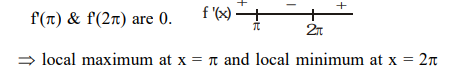# Solve this following`
Question:

For $x \in\left(0, \frac{5 \pi}{2}\right)$, define $f(x)=\int_{0}^{x} \sqrt{t} \sin t d t$. Then $f$ has :-

1. local minimum at $\pi$ and local maximum at $2 \pi$

2. local maximum at $\pi$ and local minimum at $2 \pi$

3. local maximum at $\pi$ and $2 \pi$

4. local minimum at $\pi$ and $2 \pi$

Correct Option: , 2

Solution:

$f^{\prime}(x)=\sqrt{x} \sin x$